# Open Source Bayesian Inference in Python with PyMC3¶## Who am I?¶## Bayesian Inference¶

A motivating question:

A rare disease is present in one out of one hundred thousand people. A test gives the correct diagnosis 99.9% of the time. What is the probability that a person that tests positive has the disease?

### Conditional Probability¶

Conditional probability is the probability that one event will happen, given that another event has occured.

\begin{align*} P(A\ |\ B) & = \textrm{the probability that } A \textrm{ will happen if we know that } B \textrm{ has happened} \\ & = \frac{P(A \textrm{ and } B)}{P(B)}. \end{align*}

Our question,

A rare disease is present in one out of one hundred thousand people. A test gives the correct diagnosis 99.9% of the time. What is the probability that a person that tests positive has the disease?

becomes

\begin{align*} P(+) & = 10^{-5} \\ \\ P(\textrm{Test } +\ |\ +) & = 0.999 \\ P(\textrm{Test } -\ |\ -) & = 0.999 \\ \\ P(+\ |\ \textrm{Test } +) & =\ \textbf{?} \end{align*}

### Bayes' Theorem¶

Bayes' theorem shows how to get from $P(B\ |\ A)$ to $P(A\ |\ B)$.\begin{align*} P(+\ |\ \textrm{Test } +) & = \frac{P(\textrm{Test } +\ |\ +) P(+)}{P(\textrm{Test } +)} \\ & = \frac{P(\textrm{Test } +\ |\ +) P(+)}{P(\textrm{Test } +\ |\ +) P(+) + P(\textrm{Test } +\ |\ -) P(-)} \end{align*}
\begin{align*} P(+) & = 10^{-5} \\ P(\textrm{Test } +\ |\ +) & = 0.999 \\ P(\textrm{Test } -\ |\ -) & = 0.999 \\ \\ P(+\ |\ \textrm{Test } +) & = \frac{P(\textrm{Test } +\ |\ +) P(+)}{P(\textrm{Test } +\ |\ +) P(+) + P(\textrm{Test } +\ |\ -) P(-)} \\ & = \frac{0.999 \times 10^{-5}}{0.999 \times 10^{-5} + 0.001 \times \left(1 - 10^{-5}\right)} \end{align*}
In :
0.999 * 1e-5 / (0.999 * 1e-5 + 0.001 * (1 - 1e-5))

Out:
0.009891284975940117

## Probabilistic Programming for Bayesian Inference¶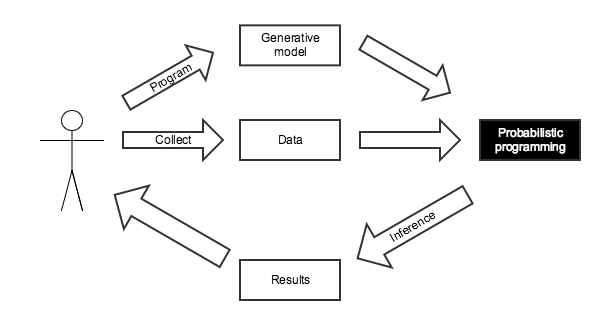We know the disease is present in one in one hundred thousand people.

In :
import pymc3 as pm

with pm.Model() as disease_model:
has_disease = pm.Bernoulli('has_disease', 1e-5)


If a person has the disease, there is a 99.9% chance they test positive. If they do not have the disease, there is a 0.1% chance they test positive.

In :
with disease_model:
p_test_pos = has_disease * 0.999 + (1 - has_disease) * 0.001


This person has tested positive for the disease.

In :
with disease_model:
test_pos = pm.Bernoulli('test_pos', p_test_pos, observed=1)


What is the probability that this person has the disease?

In :
with disease_model:
disease_trace = pm.sample(draws=10000, random_seed=SEED)

Assigned BinaryGibbsMetropolis to has_disease
100%|██████████| 10500/10500 [00:04<00:00, 2427.46it/s]

In :
disease_trace['has_disease']

Out:
array([0, 0, 0, ..., 0, 0, 0])
In :
disease_trace['has_disease'].mean()

Out:
0.0104
In :
0.999 * 1e-5 / (0.999 * 1e-5 + 0.001 * (1 - 1e-5))

Out:
0.009891284975940117

### Monte Carlo Methods¶

In :
N = 5000

x, y = np.random.uniform(0, 1, size=(2, N))

In :
fig

Out: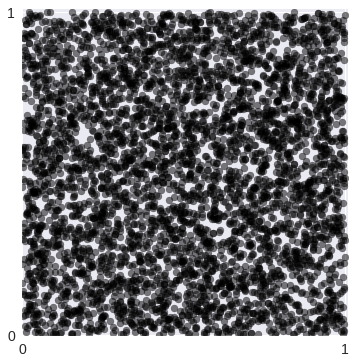In :
in_circle = x**2 + y**2 <= 1

In :
fig

Out: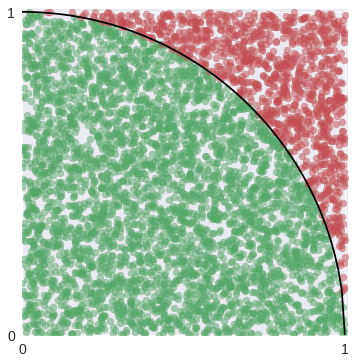In :
4 * in_circle.mean()

Out:
3.1272000000000002

#### History of Monte Carlo Methods¶## The Monty Hall Problem¶Initially, we have no information about which door the prize is behind.

In :
with pm.Model() as monty_model:
prize = pm.DiscreteUniform('prize', 0, 2)


If we choose door one:

 Monty can open Prize behind Door 1 Door 2 Door 3 Door 1 No Yes Yes Door 2 No No Yes Door 2 No Yes No
In :
from theano import tensor as tt

with monty_model:
p_open = pm.Deterministic('p_open',
tt.switch(tt.eq(prize, 0),
# it is behind the first door
np.array([0., 0.5, 0.5]),
tt.switch(tt.eq(prize, 1),
# it is behind the second door
np.array([0., 0., 1.]),
# it is behind the third door
np.array([0., 1., 0.]))))


Monty opened the third door, revealing a goat.In :
with monty_model:
opened = pm.Categorical('opened', p_open, observed=2)


Should we switch our choice of door?

In :
with monty_model:
monty_trace = pm.sample(draws=10000, random_seed=SEED)

monty_df = pm.trace_to_dataframe(monty_trace)

Assigned Metropolis to prize
100%|██████████| 10500/10500 [00:02<00:00, 4427.89it/s]

In :
monty_df.prize.head()

Out:
0    0
1    0
2    0
3    0
4    1
Name: prize, dtype: int64
In :
fig

Out: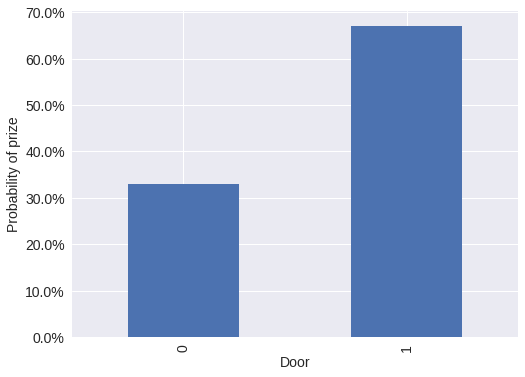Yes, we should switch our choice of door.

## Introduction to PyMC3¶From the PyMC3 documentation:

PyMC3 is a Python package for Bayesian statistical modeling and Probabilistic Machine Learning which focuses on advanced Markov chain Monte Carlo and variational fitting algorithms. Its flexibility and extensibility make it applicable to a large suite of problems.As of October 2016, PyMC3 is a NumFOCUS fiscally sponsored project.

### Features¶

• Uses Theano as a computational backend
• Automated differentiation, dynamic C compilation, GPU integration
• Implements Hamiltonian Monte Carlo and No-U-Turn sampling
• High-level GLM (R-like syntax) and Gaussian process specification
• General framework for variational inference## Case Study: Sleep Deprivation¶In :
sleep_df.head()

Out:
reaction days subject reaction_std
0 249.5600 0 308 -0.868968
1 258.7047 1 308 -0.706623
2 250.8006 2 308 -0.846944
3 321.4398 3 308 0.407108
4 356.8519 4 308 1.035777
In :
fig

Out: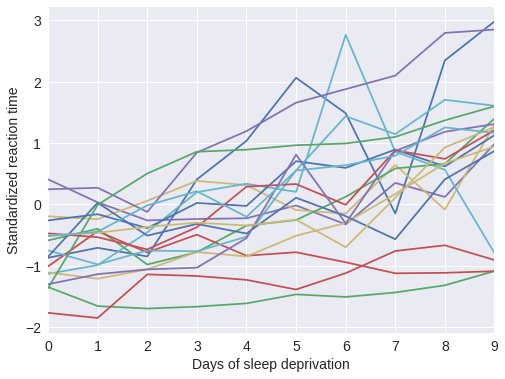Each subject has a baseline reaction time, which should not be too far apart.

In :
with pm.Model() as sleep_model:
μ_α = pm.Normal('μ_α', 0., 5.)
σ_α = pm.HalfCauchy('σ_α', 5.)
α = pm.Normal('α', μ_α, σ_α, shape=n_subjects)


Each subject's reaction time increases at a different rate after days of sleep deprivation.

In :
with sleep_model:
μ_β = pm.Normal('μ_β', 0., 5.)
σ_β = pm.HalfCauchy('σ_β', 5.)
β = pm.Normal('β', μ_β, σ_β, shape=n_subjects)


The baseline reaction time and rate of increase lead to the observed reaction times.

In :
with sleep_model:
μ = pm.Deterministic('μ', α[subject_ix] + β[subject_ix] * days)
σ = pm.HalfCauchy('σ', 5.)
obs = pm.Normal('obs', μ, σ, observed=reaction_std)

In :
N_JOBS = 3
JOB_SEEDS = [SEED + i for i in range(N_JOBS)]

with sleep_model:
sleep_trace = pm.sample(njobs=N_JOBS, random_seed=JOB_SEEDS)

Auto-assigning NUTS sampler...
Initializing NUTS using jitter+adapt_diag...
100%|██████████| 1000/1000 [00:07<00:00, 134.79it/s]


### Convergence Diagnostics¶

In :
ax = pm.energyplot(sleep_trace, legend=False)
ax.set_title("BFMI = {:.3f}".format(pm.bfmi(sleep_trace)));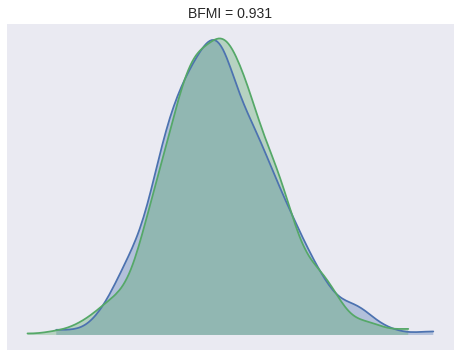In :
max(np.max(gr_values) for gr_values in pm.gelman_rubin(sleep_trace).values())

Out:
1.0019478091296214

### Prediction¶

In :
with sleep_model:
pp_sleep_trace = pm.sample_ppc(sleep_trace)

100%|██████████| 500/500 [00:00<00:00, 1258.46it/s]

In :
pp_df.head()

Out:
reaction days subject reaction_std pp_0 pp_1 pp_10 pp_100 pp_101 pp_102 ... pp_90 pp_91 pp_92 pp_93 pp_94 pp_95 pp_96 pp_97 pp_98 pp_99
0 249.5600 0 308 -0.868968 -0.221133 -1.094005 -1.479840 -0.797150 -0.251219 -1.846100 ... -0.680005 -0.036840 -0.618054 0.158745 -0.986407 -0.788178 -1.483328 -0.423254 -0.946230 -0.838087
1 258.7047 1 308 -0.706623 0.354375 -1.192640 -0.424441 0.064195 -0.661047 -1.181403 ... -0.472364 -0.825356 -0.374235 -0.336909 -0.940759 -1.236048 -0.749208 -0.892589 0.243243 -0.891522
2 250.8006 2 308 -0.846944 0.487935 -0.570185 0.304536 0.758313 0.566803 0.211791 ... -0.512914 0.156596 -0.451047 -0.219734 -0.773714 -0.237561 -0.199602 -0.071147 0.146439 -0.193735
3 321.4398 3 308 0.407108 0.950116 -0.554748 0.544648 1.037173 -0.245327 0.450455 ... -0.183340 0.289252 0.358078 1.579609 0.652802 -0.286667 -0.327654 0.607906 -0.087981 -0.097427
4 356.8519 4 308 1.035777 0.485269 0.549079 0.564043 0.045464 0.643697 0.190714 ... 0.976137 0.306833 0.744076 0.493216 0.516644 1.090693 0.583320 1.079374 0.996899 0.671175

5 rows × 504 columns

In :
grid.fig

Out: